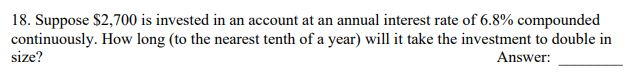# 18. Suppose \$2,700 is invested in an account at an annual interest rate of 6.8% compoundedcontinuously. How long (to the nearest tenth of a year) will it take the investment to double insize?Answer:

Question
4 views

practice question 18help_outlineImage Transcriptionclose18. Suppose \$2,700 is invested in an account at an annual interest rate of 6.8% compounded continuously. How long (to the nearest tenth of a year) will it take the investment to double in size? Answer: fullscreen
check_circle

Step 1

P0= Initial amount = 2700

r= interest rate = 6.8% =0.068

t= time = ?

P(t)= Final amount ...

### Want to see the full answer?

See Solution

#### Want to see this answer and more?

Solutions are written by subject experts who are available 24/7. Questions are typically answered within 1 hour.*

See Solution
*Response times may vary by subject and question.
Tagged in

### Algebra